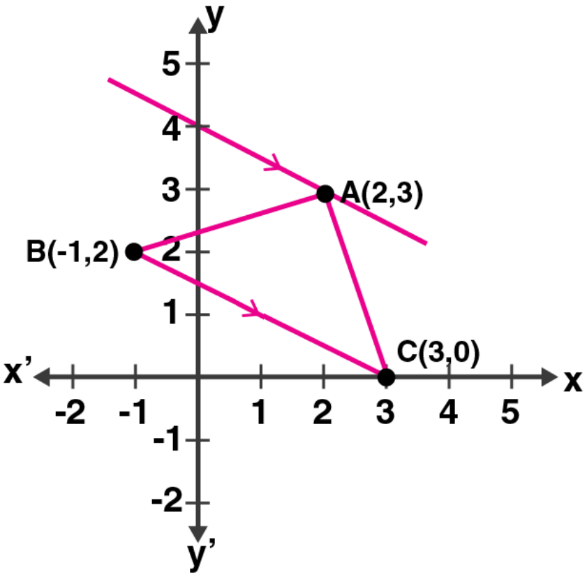Newbie

# In the adjoining diagram, write down (i) the co-ordinates of the points A, B and C. (ii) the equation of the line through A parallel to BC.

• 0

An Important Question of M.L Aggarwal book of class 10 Based on Equation of a Straight Line Chapter for ICSE BOARD.
In this question a diagram is given you have to find the co-ordinates of the points and the equation of the line through aksed as per the question.
This is the Question Number 30, Exercise 12.2 of M.L Aggarwal.

Share

1.From the given figure, its clearly seen that

Co-ordinates of A are (2, 3) and of B are (-1, 2) and of C are (3, 0).

Now,

Slope of BC = (0 – 2)/ (3 – (-1))

= -2/4

= -1/2

So, the slope of the line parallel to BC is also -1/2

And, the line passes through A (2, 3)

Hence, the equation will be

y – 3 = (-1/2) (x – 2)

2y – 6 = -x + 2

x + 2y = 8

• 0